## Mathematics at Stocksbridge Junior School

At Stocksbridge Junior School, every child is a mathematician. Our mission is to enable all learners to enjoy and succeed in Mathematics.  The value of a high-quality Mathematics education is well recognised for its importance both to the individual and to society.  Being competent in Mathematics is key for functioning within a community and being a productive part of the workforce.

The study of Mathematics reaches beyond this and ‘provides a foundation for understanding the world, the ability to reason mathematically, appreciation for the beauty and power of Mathematics, and a sense of enjoyment and curiosity about the subject.’ (National Curriculum).

Our curriculum is premised on a belief that all learners can enjoy and succeed in Mathematics, and our ultimate aim is that learners enjoy and achieve in Mathematics, developing a real interest in the subject.  Success for all is about ensuring no learner is left behind as well as ensuring more learners excel in Mathematics.  Belief that all can achieve and a commitment to providing learning experiences that allow for this are absolutely essential to accomplish success for all.  Fostering learner attitudes that are based on belief in the capacity to learn can be achieved through developing resilience and confidence.

Mathematics at Stocksbridge Junior School has been designed on principles to provide learners with a deep conceptual understanding of mathematical principles, and the ability to confidently communicate in precise mathematical language while becoming mathematical thinkers.

We believe that if a pupil understands the core principles, they will be able to remember more and do more maths, in whatever context they encounter it.

Through a wide breadth of study, we aim to ensure that pupils have a long-term memory(1) of an ambitious body of procedural(2) and semantic(3) knowledge.

## Intent

Curriculum Drivers(4) shape our curriculum breadth.  They are derived from an understanding of our pupils, our beliefs about high-quality education and our values.  They are consistently used and reviewed to ensure that we give our pupils appropriate and ambitious curriculum opportunities.

Cultural Capital(5) (developed through extending mathematical knowledge, vocabulary and experiences) gives our students the vital background knowledge required to be informed and thoughtful members of the Stocksbridge community who understand and believe in British Values.

Our curriculum distinguishes between subject topics and threshold concepts: subject topics are the specific aspects of subjects that are studied (e.g. number and place value); threshold concepts tie together the subject topics into meaningful schema(6) (see ‘Implementation’).  The same concepts are explored in all lessons.  Through this interleaving(7) of mathematical concepts across the key stage, pupils gradually build understanding of schema.

Knowledge learned within each threshold concept is built upon, and returned to, following the Mathematics Mastery progression model in order to support the building of schema.  See curriculum map.

Cognitive science research has shown that working memory is limited and cognitive load is high if pupils are rushed through content; in turn, this limits long-term memory acquisition.  Therefore, Mathematics is planned to allow pupils the time to master the basics.

## Implementation

Stocksbridge Junior School teaches Mathematics through the Mathematics Mastery curriculum which runs from Year 3 to Year 6.  The objectives taught are fully aligned with the 2014 National Curriculum programmes of study.

The programme is specifically designed to encourage learning that builds.  Key mathematical concepts are taught, applied and connected to other areas of learning throughout the year and beyond.  This enables pupils to develop their depth of understanding and mathematical fluency steadily over time in order to problem solve effectively.### The concepts of Mathematics Mastery and how they are delivered:##### Concept 1: Conceptual Understanding
1. Mathematics tasks are about constructing meaning and making sense of relationships. Learners deepen their understanding by representing concepts using objects, pictures, symbols and words.2. Different representations stress and ignore different aspects of a concept and so, moving between representations and making explicit links between them allows learners to construct a comprehensive conceptual framework that can be used as the foundation for future learning.
3. We use the content of the National Curriculum as the starting point for our curriculum, but this is expanded upon by making explicit the foundational knowledge that learners need to understand in order to access this.
4. Tasks are sequenced to help learners build a narrative through different threshold concepts. These concepts are then sequenced in a logical progression that allows learners to establish connections and draw comparisons.
5. Multiple representations are carefully selected so that they are extendable within and between different areas of Mathematics. Using these rich models encourages learners to develop different perspectives on a concept.
6. Tasks are designed so that learners are active participants and construct their own understanding of concepts (schema).
##### Concept 2: Language and Communication
1. Mathematical language strengthens conceptual understanding by enabling pupils to explain and reason.  This is carefully introduced and reinforced through frequent discussion to ensure it is meaningfully understood.
2. The more learners use mathematical words, the more they feel themselves to be mathematicians.  Talk is an essential element of every lesson and time is dedicated to developing confidence with specific vocabulary as well as verbal reasoning.
3. The content of our curriculum carefully progresses in order to induct learners into the mathematical community.  A large part of this community is confident use of the language, signs and symbols of Mathematics.  Verbal and non-verbal communication is part of every sequence of learning in the curriculum.
4. This often starts with more informal language initially, building up to formal and precise mathematical language.
5. Talk tasks are part of every lesson in the curriculum to help with this development.
##### Concept 3: Mathematical Thinking
1. By the time they reach school, all pupils have demonstrated a significant range of innate ways of thinking that can be harnessed in the classroom to develop mathematical thinking.
2. We support pupils to develop mathematical ‘habits of mind’ – to be systematic, generalise and seek out patterns.
3. The creation of a conjecturing environment and considered use of questions and prompts are important elements of encouraging learners to think like mathematicians.
4. Our curriculum is designed to give learners the opportunities to think mathematically.  Throughout the curriculum you will see tasks that require learners to specialise and generalise, to work systematically, to generate their own examples, to classify and to make conjectures.
5. This is aided by our prompts for thinking which help make these important parts of Mathematics more explicit.

### How does our mission inform the structure of our curriculum?

We have made decisions on how the curriculum is structured. These decisions are driven by the overarching principles described above and to enable all learners to enjoy and succeed in Mathematics.

#### One curriculum for all

Mathematics Mastery provides a single curriculum map that all learners are expected to follow. This means that all learners have the same access to the curriculum content and there is no ceiling imposed on what learners can achieve.

While there is only one curriculum, we recognise that not all learners come to each lesson at the same starting point.  For this reason, we provide additional resources designed to help learners access the main curriculum and also provide planning resources designed to help teachers adapt lessons to provide scaffolding and depth according to the needs of their learners.

#### A curriculum map organised into mastery half terms

In the Mathematics Mastery curriculum, extended time is spent within a single area of Mathematics.  This allows teachers to spend more time developing learners’ conceptual understanding.  It also provides opportunities to go into greater depth within a concept area and make connections with other areas of Mathematics.

#### A cumulative curriculum

The Mathematics Mastery curriculum is organised to be cumulative.  This means that mathematical concepts that are taught earlier in the curriculum are revisited in the context of a new area of Mathematics.

This helps learners to make connections between different mathematical concepts.  Retrieving, using and applying concepts regularly, transferring to new contexts helps develop fluency as well as conceptual understanding.

#### The six-part lesson structure

A six-part lesson gives a structure in which to implement the pedagogic principles of the curriculum.  The different parts of the lesson allow teachers to bring the different dimensions of depth to the foreground.  Having a consistent structure for each lesson ensures that learners are exposed to the pedagogies associated with each dimension.

### How do we support teachers to implement the curriculum?

#### Professional development

Ongoing, sustained and subject-specific professional development is at the heart of the Mathematics Mastery programme.  A greater understanding of the principles that underpin the programme will result in an enactment of the curriculum that is closer to our intention.

#### Developing subject and pedagogical knowledge

An important distinction to make when thinking about the needs of a team is between subject knowledge and pedagogical knowledge.

Professional development addresses both of these needs.  Mathematics Mastery provides opportunities for both at a variety of levels, from engaging with the lesson resources to working collaboratively with teams.

### What does assessment look like in implementation?

#### Formative assessment

Evidence points to high quality formative assessment leading to the greatest learning gains.  Planning provides opportunities and guides teachers in asking questions that will reveal learners’ understanding of a concept.  Most importantly, we provide opportunities for meaningful dialogue to take place in lessons.  It is by giving learners opportunities to talk, and by listening carefully to what they say, that we gather some of the richest data on their understanding, in order to influence teachers’ next moves.

### What does it mean to know more, remember more and be able to do more Mathematics?

In order for learners to make sense of a new idea or relationship, they need to incorporate it into their current understanding and see how it connects with ideas and relationships they have encountered previously.

The greater their understanding of what has been taught previously, the more sense-making they will be able to do in the future with increasingly complex Mathematics.  Therefore, we believe that the key to knowing more Mathematics lies in understanding.

We also believe that learners who make sense of the Mathematics they are learning have more memorable and enjoyable experiences that are more likely to be remembered in the long term.  They will also be able to do more as they understand how to push the boundaries of what they know and apply it to solve problems.

## Impact

1. Mathematics at Stocksbridge Junior School is engaging and high-quality. Our pupils embrace the Mathematics Mastery approach and develop detailed knowledge and skills across the Mathematics curriculum.
2. More importantly, pupils demonstrate a love of Mathematics and recognise its importance in the wider world. They work confidently and apply the skills that they learn to problem solving, showing outstanding learning behaviours during Mathematics lessons.
3. Pupils’ Mathematics learning is confidently transferred to, and demonstrated in, other curriculum areas.
4. As learning is a change to long-term memory, it is not practical to assess learning in the short-term. We do, however, use probabilistic assessment based on deliberate and repeated practice.  This means we look at the practices taking place to determine whether they are appropriate, related to our goals and likely to produce results in the long-run.  Practice is split into manageable parts with clear success criteria and feedback is given in the moment wherever possible.
5. We use comparative judgement in two ways: in the tasks we set and by comparing a pupil’s work over time.
6. We use lesson observations to see if the pedagogical style matches our depth expectations.
7. Pupils have many opportunities to experience mathematical learning in the outdoor environment.
8. Pupils have the understanding that Mathematics has changed our lives and that it is vital to the world’s future.
9. Pupils understand and use a richer vocabulary which enables them to articulate their understanding of mathematical concepts.
10. Pupils have high aspirations which will see them through to further study, work and a successful adult life.
11. Pupils at Stocksbridge Junior School achieve well in national tests. 2022 Key Stage 2 Mathematics SATs results.
12. Pupils leave Stocksbridge Junior School as confident and aspirational learners ready for the next stage of their Mathematics education.

## Mathematics Resources

### Times Tables

Times tables are a vital part of your child’s education and the best way to learn them is to practise, practise and then practise some more!  Pupils are expected to have secured their fluent recall of times tables by the end of Year 4.

Your child will cover times tables in class, but practising saying their tables aloud on an even more regular basis and answering quick fire recall questions is the best way for them to learn their tables by heart!

Your child will be given times tables to practise every week and will be tested on them each Friday.  Below are some suggestions that will support your child and hopefully make learning their times tables fun.

#### Methods for Learning Times Tables

Different children learn in different ways, but below are some ideas for helping your child to learn their times tables.  The most effective way is to use a combination of them all.

• Learning their tables ‘by rote’ is a traditional method, but it works well! You can help your child to learn their tables by listening to them chanting the tables in order.  You can then ask them the related division fact e.g. if they know that 6 x 3 = 18, they should be able to tell you that 18 ÷ 6 = 3 and that 18 ÷ 3 = 6

• Times table websites encourage children to apply what they have learnt and facilitate their rapid recall of multiplication facts.  Some good examples are:

Topmarks – Hit the button

Teaching Tables

Quick fire recall is an important part of learning times tables.  Children need to be able to answer questions in any order quickly (i.e. 5 seconds to answer).

Times tables facts can also be found in your child’s diary.

Further information for parents about the Year 4 Multiplication Tables Check (MTC) can be found on the DfE website.

### Useful Websites

Stocksbridge Junior School are not responsible for the content of these websites or any pop ups linked to the sites. This is a list of suggestions for websites, which have mathematical games or could be helpful with homework tasks. We advise you to check the website to ensure that you are happy that it is appropriate for your child.

## The National Curriculum for Mathematics

### Purpose of study

Mathematics is a creative and highly interconnected discipline that has been developed over centuries, providing the solution to some of history’s most intriguing problems. It is essential to everyday life, critical to science, technology and engineering, and necessary for financial literacy and most forms of employment. A high-quality mathematics education therefore provides a foundation for understanding the world, the ability to reason mathematically, an appreciation of the beauty and power of mathematics, and a sense of enjoyment and curiosity about the subject.

### Aims

The national curriculum for mathematics aims to ensure that all pupils:

• become fluent in the fundamentals of mathematics, including through varied and frequent practice with increasingly complex problems over time, so that pupils develop conceptual understanding and the ability to recall and apply knowledge rapidly and accurately
• reason mathematically by following a line of enquiry, conjecturing relationships and generalisations, and developing an argument, justification or proof using mathematical language
• can solve problems by applying their mathematics to a variety of routine and non-routine problems with increasing sophistication, including breaking down problems into a series of simpler steps and persevering in seeking solutions

Mathematics is an interconnected subject in which pupils need to be able to move fluently between representations of mathematical ideas. The programmes of study are, by necessity, organised into apparently distinct domains, but pupils should make rich connections across mathematical ideas to develop fluency, mathematical reasoning and competence in solving increasingly sophisticated problems. They should also apply their mathematical knowledge to science and other subjects.

The expectation is that the majority of pupils will move through the programmes of study at broadly the same pace. However, decisions about when to progress should always be based on the security of pupils’ understanding and their readiness to progress to the next stage. Pupils who grasp concepts rapidly should be challenged through being offered rich and sophisticated problems before any acceleration through new content. Those who are not sufficiently fluent with earlier material should consolidate their understanding, including through additional practice, before moving on.

### Information and communication technology (ICT)

Calculators should not be used as a substitute for good written and mental arithmetic. They should therefore only be introduced near the end of key stage 2 to support pupils’ conceptual understanding and exploration of more complex number problems, if written and mental arithmetic are secure. In both primary and secondary schools, teachers should use their judgement about when ICT tools should be used.

### Spoken language

The national curriculum for mathematics reflects the importance of spoken language in pupils’ development across the whole curriculum – cognitively, socially and linguistically. The quality and variety of language that pupils hear and speak are key factors in developing their mathematical vocabulary and presenting a mathematical justification, argument or proof. They must be assisted in making their thinking clear to themselves as well as others, and teachers should ensure that pupils build secure foundations by using discussion to probe and remedy their misconceptions.

### School curriculum

The programmes of study for mathematics are set out year-by-year for key stages 1 and 2. Schools are, however, only required to teach the relevant programme of study by the end of the key stage. Within each key stage, schools therefore have the flexibility to introduce content earlier or later than set out in the programme of study. In addition, schools can introduce key stage content during an earlier key stage, if appropriate. All schools are also required to set out their school curriculum for mathematics on a year-by-year basis and make this information available online.

### Lower key stage 2 – years 3 and 4

The principal focus of mathematics teaching in lower key stage 2 is to ensure that pupils become increasingly fluent with whole numbers and the 4 operations, including number facts and the concept of place value. This should ensure that pupils develop efficient written and mental methods and perform calculations accurately with increasingly large whole numbers.

At this stage, pupils should develop their ability to solve a range of problems, including with simple fractions and decimal place value. Teaching should also ensure that pupils draw with increasing accuracy and develop mathematical reasoning so they can analyse shapes and their properties, and confidently describe the relationships between them. It should ensure that they can use measuring instruments with accuracy and make connections between measure and number.

By the end of year 4, pupils should have memorised their multiplication tables up to and including the 12 multiplication table and show precision and fluency in their work.

Pupils should read and spell mathematical vocabulary correctly and confidently, using their growing word-reading knowledge and their knowledge of spelling.

### Upper key stage 2 – years 5 and 6

The principal focus of mathematics teaching in upper key stage 2 is to ensure that pupils extend their understanding of the number system and place value to include larger integers. This should develop the connections that pupils make between multiplication and division with fractions, decimals, percentages and ratio.

At this stage, pupils should develop their ability to solve a wider range of problems, including increasingly complex properties of numbers and arithmetic, and problems demanding efficient written and mental methods of calculation. With this foundation in arithmetic, pupils are introduced to the language of algebra as a means for solving a variety of problems. Teaching in geometry and measures should consolidate and extend knowledge developed in number. Teaching should also ensure that pupils classify shapes with increasingly complex geometric properties and that they learn the vocabulary they need to describe them.

By the end of year 6, pupils should be fluent in written methods for all 4 operations, including long multiplication and division, and in working with fractions, decimals and percentages.

Pupils should read, spell and pronounce mathematical vocabulary correctly.

### Number – number and place value

Pupils should be taught to:

• read, write, order and compare numbers to at least 1,000,000 and determine the value of each digit
• count forwards or backwards in steps of powers of 10 for any given number up to 1,000,000
• interpret negative numbers in context, count forwards and backwards with positive and negative whole numbers, including through 0
• round any number up to 1,000,000 to the nearest 10, 100, 1,000, 10,000 and 100,000
• solve number problems and practical problems that involve all of the above
• read Roman numerals to 1,000 (M) and recognise years written in Roman numerals

### Number – addition and subtraction

Pupils should be taught to:

• add and subtract whole numbers with more than 4 digits, including using formal written methods (columnar addition and subtraction)
• add and subtract numbers mentally with increasingly large numbers
• use rounding to check answers to calculations and determine, in the context of a problem, levels of accuracy
• solve addition and subtraction multi-step problems in contexts, deciding which operations and methods to use and why

### Number – multiplication and division

Pupils should be taught to:

• identify multiples and factors, including finding all factor pairs of a number, and common factors of 2 numbers
• know and use the vocabulary of prime numbers, prime factors and composite (non-prime) numbers
• establish whether a number up to 100 is prime and recall prime numbers up to 19
• multiply numbers up to 4 digits by a one- or two-digit number using a formal written method, including long multiplication for two-digit numbers
• multiply and divide numbers mentally, drawing upon known facts
• divide numbers up to 4 digits by a one-digit number using the formal written method of short division and interpret remainders appropriately for the context
• multiply and divide whole numbers and those involving decimals by 10, 100 and 1,000
• recognise and use square numbers and cube numbers, and the notation for squared (²) and cubed (³)
• solve problems involving multiplication and division, including using their knowledge of factors and multiples, squares and cubes
• solve problems involving addition, subtraction, multiplication and division and a combination of these, including understanding the meaning of the equals sign
• solve problems involving multiplication and division, including scaling by simple fractions and problems involving simple rates

### Number – fractions (including decimals and percentages)

Pupils should be taught to:

• compare and order fractions whose denominators are all multiples of the same number
• identify, name and write equivalent fractions of a given fraction, represented visually, including tenths and hundredths
• recognise mixed numbers and improper fractions and convert from one form to the other and write mathematical statements > 1 as a mixed number [for example,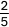+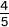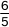= 1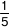]
• add and subtract fractions with the same denominator, and denominators that are multiples of the same number
• multiply proper fractions and mixed numbers by whole numbers, supported by materials and diagrams
• read and write decimal numbers as fractions [for example, 0.71 =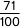]
• recognise and use thousandths and relate them to tenths, hundredths and decimal equivalents
• round decimals with 2 decimal places to the nearest whole number and to 1 decimal place
• read, write, order and compare numbers with up to 3 decimal places
• solve problems involving number up to 3 decimal places
• recognise the per cent symbol (%) and understand that per cent relates to ‘number of parts per 100’, and write percentages as a fraction with denominator 100, and as a decimal fraction
• solve problems which require knowing percentage and decimal equivalents of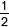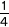and those fractions with a denominator of a multiple of 10 or 25

### Measurement

Pupils should be taught to:

• convert between different units of metric measure [for example, kilometre and metre; centimetre and metre; centimetre and millimetre; gram and kilogram; litre and millilitre]
• understand and use approximate equivalences between metric units and common imperial units such as inches, pounds and pints
• measure and calculate the perimeter of composite rectilinear shapes in centimetres and metres
• calculate and compare the area of rectangles (including squares), including using standard units, square centimetres (cm²) and square metres (m²), and estimate the area of irregular shapes
• estimate volume [for example, using 1 cm³ blocks to build cuboids (including cubes)] and capacity [for example, using water]
• solve problems involving converting between units of time
• use all four operations to solve problems involving measure [for example, length, mass, volume, money] using decimal notation, including scaling

### Geometry – properties of shapes

Pupils should be taught to:

• identify 3-D shapes, including cubes and other cuboids, from 2-D representations
• know angles are measured in degrees: estimate and compare acute, obtuse and reflex angles
• draw given angles, and measure them in degrees (°)
• identify:
• angles at a point and 1 whole turn (total 360°)
• angles at a point on a straight line and half a turn (total 180°)
• other multiples of 90°
• use the properties of rectangles to deduce related facts and find missing lengths and angles
• distinguish between regular and irregular polygons based on reasoning about equal sides and angles

### Geometry – position and direction

Pupils should be taught to:

• identify, describe and represent the position of a shape following a reflection or translation, using the appropriate language, and know that the shape has not changed

### Statistics

Pupils should be taught to:

• solve comparison, sum and difference problems using information presented in a line graph
• complete, read and interpret information in tables, including timetables

### Number – number and place value

Pupils should be taught to:

• read, write, order and compare numbers up to 10,000,000 and determine the value of each digit
• round any whole number to a required degree of accuracy
• use negative numbers in context, and calculate intervals across 0
• solve number and practical problems that involve all of the above

### Number – addition, subtraction, multiplication and division

Pupils should be taught to:

• multiply multi-digit numbers up to 4 digits by a two-digit whole number using the formal written method of long multiplication
• divide numbers up to 4 digits by a two-digit whole number using the formal written method of long division, and interpret remainders as whole number remainders, fractions, or by rounding, as appropriate for the context
• divide numbers up to 4 digits by a two-digit number using the formal written method of short division where appropriate, interpreting remainders according to the context
• perform mental calculations, including with mixed operations and large numbers
• identify common factors, common multiples and prime numbers
• use their knowledge of the order of operations to carry out calculations involving the 4 operations
• solve addition and subtraction multi-step problems in contexts, deciding which operations and methods to use and why
• solve problems involving addition, subtraction, multiplication and division
• use estimation to check answers to calculations and determine, in the context of a problem, an appropriate degree of accuracy

### Number – Fractions (including decimals and percentages)

Pupils should be taught to:

• use common factors to simplify fractions; use common multiples to express fractions in the same denomination
• compare and order fractions, including fractions >1
• add and subtract fractions with different denominators and mixed numbers, using the concept of equivalent fractions
• multiply simple pairs of proper fractions, writing the answer in its simplest form [for example,×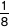]
• divide proper fractions by whole numbers [for example,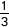÷ 2 =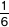]
• associate a fraction with division and calculate decimal fraction equivalents [for example, 0.375] for a simple fraction [for example,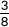]
• identify the value of each digit in numbers given to 3 decimal places and multiply and divide numbers by 10, 100 and 1,000 giving answers up to 3 decimal places
• multiply one-digit numbers with up to 2 decimal places by whole numbers
• use written division methods in cases where the answer has up to 2 decimal places
• solve problems which require answers to be rounded to specified degrees of accuracy
• recall and use equivalences between simple fractions, decimals and percentages, including in different contexts

### Ratio and proportion

Pupils should be taught to:

• solve problems involving the relative sizes of 2 quantities where missing values can be found by using integer multiplication and division facts
• solve problems involving the calculation of percentages [for example, of measures and such as 15% of 360] and the use of percentages for comparison
• solve problems involving similar shapes where the scale factor is known or can be found
• solve problems involving unequal sharing and grouping using knowledge of fractions and multiples

### Algebra

Pupils should be taught to:

• use simple formulae
• generate and describe linear number sequences
• express missing number problems algebraically
• find pairs of numbers that satisfy an equation with 2 unknowns
• enumerate possibilities of combinations of 2 variables

### Measurement

Pupils should be taught to:

• solve problems involving the calculation and conversion of units of measure, using decimal notation up to 3 decimal places where appropriate
• use, read, write and convert between standard units, converting measurements of length, mass, volume and time from a smaller unit of measure to a larger unit, and vice versa, using decimal notation to up to 3 decimal places
• convert between miles and kilometres
• recognise that shapes with the same areas can have different perimeters and vice versa
• recognise when it is possible to use formulae for area and volume of shapes
• calculate the area of parallelograms and triangles
• calculate, estimate and compare volume of cubes and cuboids using standard units, including cubic centimetres (cm³) and cubic metres (m³), and extending to other units [for example, mm³ and km³]

### Geometry – properties of shapes

Pupils should be taught to:

• draw 2-D shapes using given dimensions and angles
• recognise, describe and build simple 3-D shapes, including making nets
• compare and classify geometric shapes based on their properties and sizes and find unknown angles in any triangles, quadrilaterals, and regular polygons
• illustrate and name parts of circles, including radius, diameter and circumference and know that the diameter is twice the radius
• recognise angles where they meet at a point, are on a straight line, or are vertically opposite, and find missing angles

### Geometry – position and direction

Pupils should be taught to:

• describe positions on the full coordinate grid (all 4 quadrants)
• draw and translate simple shapes on the coordinate plane, and reflect them in the axes

### Statistics

Pupils should be taught to:

• interpret and construct pie charts and line graphs and use these to solve problems
• calculate and interpret the mean as an average

### Number – number and place value

Pupils should be taught to:

• count from 0 in multiples of 4, 8, 50 and 100; find 10 or 100 more or less than a given number
• recognise the place value of each digit in a 3-digit number (100s, 10s, 1s)
• compare and order numbers up to 1,000
• identify, represent and estimate numbers using different representations
• read and write numbers up to 1,000 in numerals and in words
• solve number problems and practical problems involving these ideas

### Number – addition and subtraction

Pupils should be taught to:

• add and subtract numbers mentally, including:
• a three-digit number and 1s
• a three-digit number and 10s
• a three-digit number and 100s
• add and subtract numbers with up to 3 digits, using formal written methods of columnar addition and subtraction
• estimate the answer to a calculation and use inverse operations to check answers
• solve problems, including missing number problems, using number facts, place value, and more complex addition and subtraction

### Number – multiplication and division

Pupils should be taught to:

• recall and use multiplication and division facts for the 3, 4 and 8 multiplication tables
• write and calculate mathematical statements for multiplication and division using the multiplication tables that they know, including for two-digit numbers times one-digit numbers, using mental and progressing to formal written methods
• solve problems, including missing number problems, involving multiplication and division, including positive integer scaling problems and correspondence problems in which n objects are connected to m objects

### Number – fractions

Pupils should be taught to:

• count up and down in tenths; recognise that tenths arise from dividing an object into 10 equal parts and in dividing one-digit numbers or quantities by 10
• recognise, find and write fractions of a discrete set of objects: unit fractions and non-unit fractions with small denominators
• recognise and use fractions as numbers: unit fractions and non-unit fractions with small denominators
• recognise and show, using diagrams, equivalent fractions with small denominators
• add and subtract fractions with the same denominator within one whole [for example,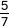+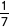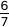]
• compare and order unit fractions, and fractions with the same denominators
• solve problems that involve all of the above

### Measurement

Pupils should be taught to:

• measure, compare, add and subtract: lengths (m/cm/mm); mass (kg/g); volume/capacity (l/ml)
• measure the perimeter of simple 2-D shapes
• add and subtract amounts of money to give change, using both £ and p in practical contexts
• tell and write the time from an analogue clock, including using Roman numerals from I to XII, and 12-hour and 24-hour clocks
• estimate and read time with increasing accuracy to the nearest minute; record and compare time in terms of seconds, minutes and hours; use vocabulary such as o’clock, am/pm, morning, afternoon, noon and midnight
• know the number of seconds in a minute and the number of days in each month, year and leap year
• compare durations of events [for example, to calculate the time taken by particular events or tasks]

### Geometry – properties of shapes

Pupils should be taught to:

• draw 2-D shapes and make 3-D shapes using modelling materials; recognise 3-D shapes in different orientations and describe them
• recognise angles as a property of shape or a description of a turn
• identify right angles, recognise that 2 right angles make a half-turn, 3 make three-quarters of a turn and 4 a complete turn; identify whether angles are greater than or less than a right angle
• identify horizontal and vertical lines and pairs of perpendicular and parallel lines

### Statistics

Pupils should be taught to:

• interpret and present data using bar charts, pictograms and tables
• solve one-step and two-step questions [for example ‘How many more?’ and ‘How many fewer?’] using information presented in scaled bar charts and pictograms and tables

### Number – number and place value

Pupils should be taught to:

• count in multiples of 6, 7, 9, 25 and 1,000
• find 1,000 more or less than a given number
• count backwards through 0 to include negative numbers
• recognise the place value of each digit in a four-digit number (1,000s, 100s, 10s, and 1s)
• order and compare numbers beyond 1,000
• identify, represent and estimate numbers using different representations
• round any number to the nearest 10, 100 or 1,000
• solve number and practical problems that involve all of the above and with increasingly large positive numbers
• read Roman numerals to 100 (I to C) and know that over time, the numeral system changed to include the concept of 0 and place value

### Number – addition and subtraction

Pupils should be taught to:

• add and subtract numbers with up to 4 digits using the formal written methods of columnar addition and subtraction where appropriate
• estimate and use inverse operations to check answers to a calculation
• solve addition and subtraction two-step problems in contexts, deciding which operations and methods to use and why

### Number – multiplication and division

Pupils should be taught to:

• recall multiplication and division facts for multiplication tables up to 12 × 12
• use place value, known and derived facts to multiply and divide mentally, including: multiplying by 0 and 1; dividing by 1; multiplying together 3 numbers
• recognise and use factor pairs and commutativity in mental calculations
• multiply two-digit and three-digit numbers by a one-digit number using formal written layout
• solve problems involving multiplying and adding, including using the distributive law to multiply two-digit numbers by 1 digit, integer scaling problems and harder correspondence problems such as n objects are connected to m objects

### Number – fractions (including decimals)

Pupils should be taught to:

• recognise and show, using diagrams, families of common equivalent fractions
• count up and down in hundredths; recognise that hundredths arise when dividing an object by 100 and dividing tenths by 10
• solve problems involving increasingly harder fractions to calculate quantities, and fractions to divide quantities, including non-unit fractions where the answer is a whole number
• add and subtract fractions with the same denominator
• recognise and write decimal equivalents of any number of tenths or hundreds
• recognise and write decimal equivalents to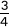• find the effect of dividing a one- or two-digit number by 10 and 100, identifying the value of the digits in the answer as ones, tenths and hundredths
• round decimals with 1 decimal place to the nearest whole number
• compare numbers with the same number of decimal places up to 2 decimal places
• solve simple measure and money problems involving fractions and decimals to 2 decimal places

### Measurement

Pupils should be taught to:

• convert between different units of measure [for example, kilometre to metre; hour to minute]
• measure and calculate the perimeter of a rectilinear figure (including squares) in centimetres and metres
• find the area of rectilinear shapes by counting squares
• estimate, compare and calculate different measures, including money in pounds and pence
• read, write and convert time between analogue and digital 12- and 24-hour clocks
• solve problems involving converting from hours to minutes, minutes to seconds, years to months, weeks to days

### Geometry – properties of shapes

Pupils should be taught to:

• compare and classify geometric shapes, including quadrilaterals and triangles, based on their properties and sizes
• identify acute and obtuse angles and compare and order angles up to 2 right angles by size
• identify lines of symmetry in 2-D shapes presented in different orientations
• complete a simple symmetric figure with respect to a specific line of symmetry

### Geometry – position and direction

Pupils should be taught to:

• describe positions on a 2-D grid as coordinates in the first quadrant
• describe movements between positions as translations of a given unit to the left/right and up/down
• plot specified points and draw sides to complete a given polygon

### Statistics

Pupils should be taught to:

• interpret and present discrete and continuous data using appropriate graphical methods, including bar charts and time graphs
• solve comparison, sum and difference problems using information presented in bar charts, pictograms, tables and other graphs

1Long-term memory – learning is a change to long-term memory; something in a person’s long-term memory has been learned.

2Procedural knowledge – knowing how to do something.

3Semantic knowledge – basic facts acquired over time (e.g. 5 + 5 = 10).

4Curriculum Drivers – factors that have influenced how we have planned our curriculum; these are based on the backgrounds of our pupils, our beliefs and our values.5

5Cultural Capital – the background knowledge of the world that pupils need for inference and understanding.

6Schema – how individual groups of knowledge are interconnected; schema help pupils organise and interpret information.

7Interleaving – mixing the learning of threshold concepts to support long-term memory.